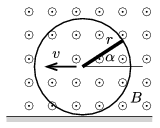Mathematical and Physical Journal
for High Schools
Issued by the MATFUND Foundation
 Already signed up? New to KöMaL?

#Problem P. 4828. (March 2016)

P. 4828. The disc if radius $\displaystyle r$, shown in the figure is made of some insulating material, and is rolling in uniform magnetic field without slipping along the horizontal ground at a constant speed of $\displaystyle v$. The magnetic induction $\displaystyle B$ is perpendicular to the plane of the disc. A thin copper rod of radius $\displaystyle r$ joins the centre of the disc with the rim of the disc. Plot the induced electromotive force between the two ends of the copper rod as a function of the angle $\displaystyle \alpha$, which is measured between the rod and the horizontal.(4 pont)

Deadline expired on April 11, 2016.

### Statistics:

 30 students sent a solution. 4 points: Bartók Imre, Bekes Nándor, Csire Roland, Csorba Benjámin, Csuha Boglárka, Fehér 169 Szilveszter, Forrai Botond, Iván Balázs, Kasza Bence, Kovács Péter Tamás, Németh 777 Róbert, Sal Kristóf, Szentivánszki Soma , Takács Attila, Zöllner András. 3 points: Asztalos Bogdán, Csenger Géza, Jakus Balázs István, Körmöczi Dávid, Németh Flóra Boróka, Pázmán Előd, Radnai Bálint, Szántó Benedek, Tófalusi Ádám, Varga-Umbrich Eszter. 2 points: 3 students. 1 point: 1 student. 0 point: 1 student.

Problems in Physics of KöMaL, March 2016